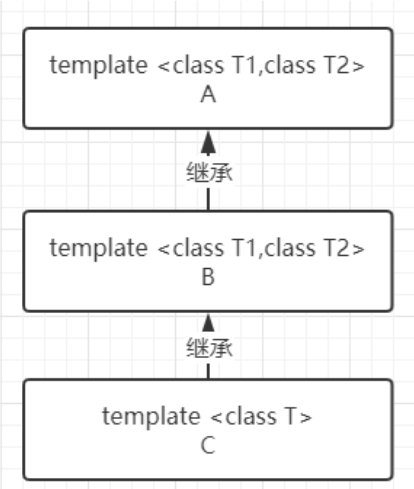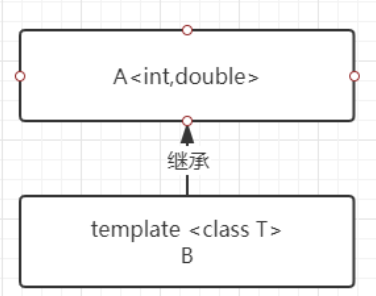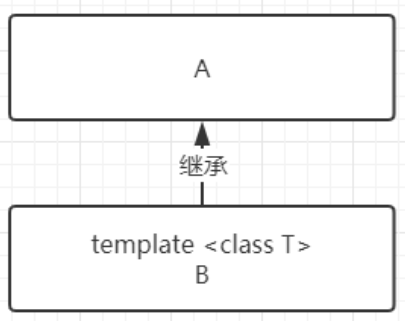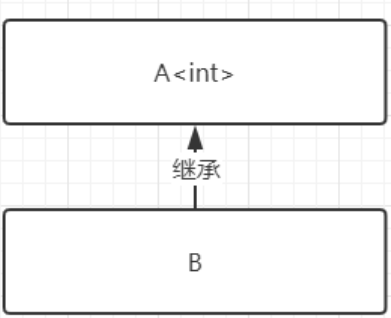# C++ 模板常见特性（函数模板、类模板）

0收藏

C++ 是很强大，有各种特性来提高代码的可重用性，有助于减少开发的代码量和工作量。

C++ 提高代码的可重用性主要有两方面：

• 继承
• 模板

01 变量交换函数模板

``````// 交换两个整型变量的值的Swap函数：
void Swap(int & x,int & y)
{
int tmp = x;
x = y;
y = tmp;
}``````

``````// 交换两个double型变量的值的Swap函数:
void Swap(double & x,double & y)
{
double tmp = x;
x = y;
y = tmp;
}``````

``````template <class 类型参数1，class 类型参数2，...>

{
函数体
};``````

template 就是模板定义的关键词，T 代表的是任意变量的类型。

``````template <class T>
void Swap(T & x,T & y)
{
T tmp = x;
x = y;
y = tmp;
}``````

``````int main()
{
int n = 1,m = 2;
Swap(n,m); //编译器自动生成 void Swap(int & ,int & )函数

double f = 1.2,g = 2.3;
Swap(f,g); //编译器自动生成 void Swap(double & ,double & )函数

return 0;
}``````

``````int main()
{
int n = 1,m = 2;
Swap<int>(n,m);     // 指定模板函数的变量类型为int

double f = 1.2,g = 2.3;
Swap<double>(f,g); // 指定模板函数的变量类型为double

return 0;
}
``````

02 查询数组最大值函数模板

``````// 求数组最大元素的MaxElement函数模板
template <class T>
T MaxElement(T a[], int size) // size是数组元素个数
{
T tmpMax = a;
for(int i = 1;i < size;++i)
{
if(tmpMax < a[i])
{
tmpMax = a[i];
}
}
return tmpMax;
}``````

03 多个类型参数模板函数

``````template <class T1, class T2>
T2 MyFun(T1 arg1, T2 arg2)
{
cout<< arg1 << " "<< arg2<<endl;
return arg2;
}``````

T1 是传入的第一种任意变量类型，T2 是传入的第二种任意变量类型。

04 函数模板的重载

``````// 模板函数 1
template<class T1, class T2>
void print(T1 arg1, T2 arg2)
{
cout<< arg1 << " "<< arg2<<endl;
}

// 模板函数 2
template<class T>
void print(T arg1, T arg2)
{
cout<< arg1 << " "<< arg2<<endl;
}

// 模板函数 3
template<class T,class T2>
void print(T arg1, T arg2)
{
cout<< arg1 << " "<< arg2<<endl;
}``````

05 函数模板和函数的次序

1. 先找参数完全匹配的普通函数（非由模板实例化而得的函数）；
2. 再找参数完全匹配的模板函数；
3. 再找实参数经过自动类型转换后能够匹配的普通函数；
4. 上面的都找不到，则报错。

``````// 模板函数 - 1个参数类型
template <class T>
T Max(T a, T b)
{
cout << "TemplateMax" <<endl; return 0;
}

// 模板函数 - 2个参数类型
template <class T, class T2>
T Max(T a, T2 b)
{
cout << "TemplateMax2" <<endl; return 0;
}

// 普通函数
double Max(double a, double b)
{
cout << "MyMax" << endl;
return 0;
}

int main()
{
int i=4, j=5;

// 输出MyMax - 匹配普通函数
Max( 1.2, 3.4 );

//输出TemplateMax - 匹配参数一样的模板函
Max( i, j );

//输出TemplateMax2 - 匹配参数类型不同的模板函数
Max( 1.2, 3 );

return 0;
}``````

``````// 模板函数 - 1个参数类型
template<class T>
T myFunction( T arg1, T arg2)
{
cout<<arg1<<" "<<arg2<<"\n";
return arg1;
}

...

// OK ：替换 T 为 int 类型
myFunction( 5, 7);

// OK ：替换 T 为 double 类型
myFunction(5.8, 8.4);

// error ：没有匹配到myFunction(int, double)函数
myFunction(5, 8.4); ``````

01 类模板的定义

``````template <class 类型参数1，class 类型参数2，...> //类型参数表
class 类模板名
{
成员函数和成员变量
};``````

``类模板名<真实类型参数表> 对象名(构造函数实参表);``

02 Pair类模板例子

``````// 类模板
template <class T1, class T2>
class Pair
{
public:
Pair(T1 k, T2 v):m_key(k),m_value(v) {};
bool operator < (const Pair<T1,T2> & p) const;
private:
T1 m_key;
T2 m_value;
};

// 类模板里成员函数的写法
template <class T1, class T2>
bool Pair<T1,T2>::operator < (const Pair<T1,T2> &p) const
{
return m_value < p.m_value;
}

int main()
{
Pair<string,int> Astudent("Jay",20);
Pair<string,int> Bstudent("Tom",21);

cout << (Astudent < Bstudent) << endl;

return 0;
}``````

``1``

``````Pair<string,int> *p;
Pair<string,double> a;
p = & a; //错误！！``````

03 函数模板作为类模板成员

``````// 类模板
template <class T>
class A
{
public:
template<class T2>
void Func(T2 t) { cout << t; } // 成员函数模板
};

int main()
{
A<int> a;
a.Func('K');     //成员函数模板 Func被实例化
a.Func("hello"); //成员函数模板 Func再次被实例化

return 0;
} ``````

04 类模板与非类型参数

``````template <class T, int size>
class CArray
{
public:
void Print( )
{
for( int i = 0;i < size; ++i)
cout << array[i] << endl;
}
private:
T array[size];
};

CArray<double,40> a2;
CArray<int,50> a3; //a2和a3属于不同的类``````

01 类模板从类模板派生``````// 基类 - 类模板
template <class T1,class T2>
class A
{
T1 v1; T2 v2;
};

// 派生类 - 类模板
template <class T1,class T2>
class B:public A<T2,T1>
{
T1 v3; T2 v4;
};

// 派生类 - 类模板
template <class T>
class C:public B<T,T>
{
T v5;
};

int main()
{
B<int,double> obj1;
C<int> obj2;
return 0;
}``````

02 类模板从模板类派生``````template <class T1,class T2>
class A
{
T1 v1; T2 v2;
};

template <class T>
class B:public A<int,double>  // A<int,double> 模板类
{
T v;
};

int main()
{
//自动生成两个模板类 ：A<int,double> 和 B<char>
B<char> obj1;
return 0;
}``````

03 类模板从普通类派生``````// 基类 - 普通类
class A
{
int v1;
};

// 派生类 - 类模板
template <class T>
class B:public A  // 所有从B实例化得到的类 ，都以A为基类
{
T v;
};

int main()
{
B<char> obj1;
return 0;
}``````

04 普通类从模板类派生``````template <class T>
class A
{
T v1;
};

class B:public A<int>
{
double v;
};

int main()
{
B obj1;
return 0;
}``````

01 函数、类、类的成员函数作为类模板的友元

``````// 普通函数
void Func1() { }

// 普通类
class A { };

// 普通类
class B
{
public:
void Func() { } // 成员函数
};

// 类模板
template <class T>
class Tmp
{
friend void Func1();    // 友元函数
friend class A;         // 友元类
friend void B::Func();  // 友元类的成员函数
}; // 任何从 Tmp 实例化来的类 ，都有以上三个友元``````

02 函数模板作为类模板的友元

``````// 类模板
template <class T1,class T2>
class Pair
{
private:
T1 key;   //关键字
T2 value; //值
public:
Pair(T1 k,T2 v):key(k),value(v) { };

// 友元函数模板
template <class T3,class T4>
friend ostream & operator<< (ostream & o, const Pair<T3,T4> & p);
};

// 函数模板
template <class T3,class T4>
ostream & operator<< (ostream & o, const Pair<T3,T4> & p)
{
o << "(" << p.key << "," << p.value << ")" ;
return o;
}

int main()
{
Pair<string,int> student("Tom",29);
Pair<int,double> obj(12,3.14);

cout << student << " " << obj;
return 0;
}``````

``(Tom,29) (12,3.14)``

03 函数模板作为类的友元

``````// 普通类
class A
{
private:
int v;
public:
A(int n):v(n) { }

template <class T>
friend void Print(const T & p); // 函数模板
};

// 函数模板
template <class T>
void Print(const T & p)
{
cout << p.v;
}

int main()
{
A a(4);
Print(a);
return 0;
}``````

``4``

04 类模板作为类模板的友元

``````// 类模板
template <class T>
class B
{
private:
T v;
public:
B(T n):v(n) { }

template <class T2>
friend class A; // 友元类模板
};

// 类模板
template <class T>
class A
{
public:
void Func( )
{
B<int> o(10); // 实例化B模板类
cout << o.v << endl;
}
};

int main()
{
A<double> a;
a.Func ();
return 0;
}``````

``10``

``````template <class T>
class A
{
private:
static int count; // 静态成员
public:
A() { count ++; }
~A() { count -- ; };
A( A & ) { count ++ ; }

static void PrintCount() { cout << count << endl; } // 静态函数
};

template<> int A<int>::count = 0;    // 初始化
template<> int A<double>::count = 0; // 初始化

int main()
{
A<int> ia;
A<double> da; // da和ia不是相同模板类
ia.PrintCount();
da.PrintCount();
return 0;
}``````

``````1
1``````

• 类模板里的静态成员初始化的时候，最前面要加template<>。
• ia 和 da 对象是不同的模板类，因为类型参数是不一致，所以也就是不同的模板类。帖子
视频
声望
粉丝
社区精华内容